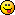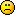#### Topic: moving average rise in the indicator HTF

Hello
I usually make a "moving rise" by writing:
shift 1 > shift 2.

in the indicator HTF, the setup is different..
It is better because it is more selective, I think.
But I do not understand!
" (hfClose[iBar - iFrame] < dValue && dValue < hfOpen[iBar])|| // Positive gap "
Can someone explain me?
thanks
CORSICA#### Re: moving average rise in the indicator HTF

iBar = this bar, the current bar you're looking at

iFrame = an adjustment value to go from your chart's time frame (ex, 5 minutes) to the Higher Time Frame (ex, 60 minutes), given as (higher time frame in minutes) / (current time frame in minutes)
Ex: current time frame = 5 minutes, higher time frame = 60, so iFrame = 60/5 = 12
meaning, Higher Time Frame indicator changes over 12 bars instead of 1 bar

dValue = MA value at this bar
hfOpen[iBar] = open price of the current bar
hfClose[iBar-iFrame] = close price of bar higher time frame bars ago (see iFrame above)

so this line:
(hfClose[iBar - iFrame] < dValue && dValue < hfOpen[iBar])|| // Positive gap

means "if the close from higher time frame bars ago is less than the MA value, AND the current open is higher than the MA value, THEN do something"

the "// Positive gap" is not part of the code,the author makes a note this case is where the price gaps upwards. But the "||" is an OR, it looks like he has 4 different possible conditions for setting the entry price of the position.

But be sure to test using these indicators on FSB and FST in practice or demo accounts before using them, there have been quite a few posts that the HTF (Higher Time Frame) indicators have some bugs where the FST trade executions don't match the FSB backtesting.

hope that helps

#### Re: moving average rise in the indicator HTF

thank you verry much to KROG

#### Re: moving average rise in the indicator HTF

To KROG
below the formula in the language MQ4 as I write and it is not good
void TechnicalAnalysis38() {
if (iMA(NULL, PERIOD_M5, 29, 0, MODE_LWMA, PRICE_CLOSE, 1) > iMA(NULL, PERIOD_M5, 29, 0, MODE_LWMA, PRICE_CLOSE, 2)) TechnicalAnalysis31();

how to write the formula in the language MQ4.
(hfClose[iBar - iFrame] < dValue && dValue < hfOpen[iBar])|| // Positive gap

sorry, I'm a bit long to understand

#### Re: moving average rise in the indicator HTF

below the formula in the language MQ4 as I write and it is not good
void TechnicalAnalysis38() {
if (iMA(NULL, PERIOD_M5, 29, 0, MODE_LWMA, PRICE_CLOSE, 1) > iMA(NULL, PERIOD_M5, 29, 0, MODE_LWMA, PRICE_CLOSE, 2)) TechnicalAnalysis31();

how to write the formula in the language MQ4.
(hfClose[iBar - iFrame] < dValue && dValue < hfOpen[iBar])|| // Positive gap

sorry, I'm a bit long to understand

#### Re: moving average rise in the indicator HTF

I'm sorry -- I don't know MQ4 enough to answer. It would take a lot of work to add the "higher time frame" concept to MQ4 indicators (but maybe someone has done it already).

But I don't think your MQ4 line above is the same; it looks like comparing 2 MA values, the previous MA value and the current MA value.
The HTF MA is comparing the MA value against the previous price close (on higher time frame) against the current price open (on higher time frame).

Sorry I couldn't help more.ok, KROG
thank you